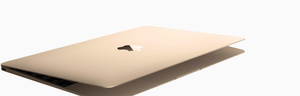# Refurbished Mac

Discover what goes into each refurbished Mac.

A\$929.00

A\$969.00

A\$1,099.00

A\$1,149.00

A\$1,149.00

A\$1,189.00

A\$1,209.00

A\$1,269.00

A\$1,319.00

A\$1,349.00

A\$1,359.00

A\$1,359.00

A\$1,359.00

A\$1,359.00

A\$1,409.00

A\$1,429.00

A\$1,429.00

A\$1,429.00

A\$1,459.00

A\$1,489.00

A\$1,589.00

A\$1,599.00

A\$1,609.00

A\$1,609.00

A\$1,609.00

A\$1,659.00

A\$1,659.00

A\$1,679.00

A\$1,679.00

A\$1,679.00

A\$1,699.00

A\$1,729.00

A\$1,729.00

A\$1,739.00

A\$1,859.00

A\$1,859.00

A\$1,869.00

A\$1,869.00

A\$1,929.00

A\$1,929.00

A\$1,929.00

A\$1,949.00

A\$1,949.00

A\$1,949.00

A\$1,949.00

A\$1,999.00

A\$1,999.00

A\$2,119.00

A\$2,119.00

A\$2,189.00

A\$2,209.00

A\$2,209.00

A\$2,289.00

A\$2,359.00

A\$2,459.00

A\$2,459.00

A\$2,549.00

A\$2,549.00

A\$2,679.00

A\$2,699.00

A\$2,699.00

A\$2,799.00

A\$2,799.00

A\$2,799.00

A\$2,799.00

A\$2,919.00

A\$2,919.00

A\$2,969.00

A\$2,969.00

A\$3,059.00

A\$3,059.00

A\$3,069.00

A\$3,229.00

A\$3,229.00

A\$3,569.00

A\$3,569.00

A\$3,569.00

A\$3,569.00

A\$3,739.00

A\$3,739.00

A\$3,769.00

A\$3,769.00

A\$3,879.00

A\$3,879.00

A\$4,049.00

A\$4,049.00

A\$4,079.00

A\$4,079.00

A\$4,279.00

A\$4,279.00

A\$4,419.00

A\$4,419.00

A\$4,419.00

A\$4,529.00

A\$4,529.00

A\$4,669.00

A\$4,729.00

A\$4,829.00

A\$4,829.00

A\$4,839.00

A\$4,959.00

A\$5,209.00

A\$5,209.00

A\$5,369.00

A\$5,399.00

A\$5,509.00

A\$5,759.00

A\$5,759.00

A\$5,779.00

A\$5,909.00

A\$5,909.00

A\$6,029.00

A\$6,159.00

A\$6,319.00

A\$6,439.00

A\$6,569.00

A\$6,569.00

A\$7,179.00

A\$7,179.00

A\$8,199.00

A\$8,459.00

A\$8,709.00